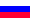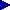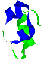Biochemistry-online­¾±±ĻĶķ
 home >> biochemistry-online >> calculation algorithm last updated 16.01.04

calcualtion algorithm

calculation examples

authors

 Quick calculation
put here aminoacid sequence and press button

for the more options use main calculation pageCharge at fixed pH.

This function is also used when the isoelectric point is being calculated. The charge of protein molecule is a result of acid-base equilibria, which occur in water solution. There are two types of this equilibrium which can take place:

1) Protonisation of uncharged group (e.g. amides)

AH+ = A + H+
This group brings positive contribution to the total charge of the molecule. The contribution is equal to the part of charged groups from the total number of the groups:
q = [AH+]/([AH+]+[A]) = 1/(1 + [A]/[AH+])
One can get the value [A]/[AH+] from the dissociation constant expression:
K = ([H+]*[A])/[AH+]
Hence:
q = 1/(1 + 10(pH-pK))

2) Dissociation of uncharged group (e.g. carboxylic)
BH = B- + H+
Group of this type contributes negative charge, which is calculated in the same way:
q = -[B-]/([BH]+[B-]) = -1/(1 + [BH]/[B-])
q = -1/(1 + 10(pK-pH))

The total charge is the sum of all group charges:
Q(pH) = q1 + ... + qn
When the charge of given protein is calculated, all side groups, N and C-terminal groups (amides and carbosyl) are considered. Dissociation constant values are taken from the "Chemistry encyclopedia" edited I.L.Kunyantz, Moscow, 1998.Isoelectric point.

To calculate the isoelectric point one should solve follows equation
Q(pH) = 0

The function Q(pH) has different signes at pH = 0 and pH = 14, so one can use the dichotomy method to solve this equation.Molecular weight.

First, the brutto-formula of the protein is written. Then the molecular weigth is calculated taking isotope content into account. If "isotope" options are not chosen, the natural isotope content is used.
When the molecular weight is calculated the dissociation/protonisation of functional group is not considered. To correct the results one should compute the total charge of molecule which is in the direct connection with the number of associated/dissociated protons.Calculation error.

The results of calculation (particularly isoelectric point and total charge) contain several error because of the difference between real and theoretical dissociation constants. Some facts cause this difference. First of all the charge of protein molecule affects the dissociation/protonisation processes (it can both reduce and increase constants). Secondly the protein molecule 3-D structure is not taken into account. Protein molecule is folded, and the charge is not distributed constantly because of the shielding of some functional groups.
So obtained results should be used only as an estimation of real values. You can see the results of testing of the program on different protein molecules.
If you have some questions, mail me vit-send@yandex.ru

UP